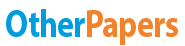# Chemistry Homework

Essay by   •  September 23, 2012  •  Study Guide  •  367 Words (2 Pages)  •  3,959 Views

## Essay Preview: Chemistry Homework

1 rating(s)
Report this essay
Page 1 of 2

Homework #1

How does a hypothesis differ from a theory?

1) A hypothesis is a reasonable and testable explanation for observations. A theory, on the other hand, is a well-tested explanation of observations.

What is the scientific method?

2) The scientific method is a series of steps followed to solve problems, including collecting data, formulating a hypothesis, testing the hypothesis, and stating conclusions.

Do experiments always turn out as expected? Why or why not?

3) No experiments do not always turn out as expected. Scientists' results often don't match their initial predictions. Instead of viewing this as a failure scientists analyze the results and continue on with the scientific method. Unexpected results are as important as expected results because they both provide an equal amount of information.

What is a scientific law, and how does it differ from a theory?

4) A theory is an explanation for some phenomenon that is based on observation, experimentation, and reasoning. A law is a summary of many experimental results and observations; a law tells us how something works.

Why does a scientist include a control in the design of an experiment?

5) When a variable is kept constant from one experiment to the next, the variable is called a control and the procedure is called a controlled experiment. Controls are used to determine if some factor that is not being considered is responsible for the changes your hypothesis.

Why is there no single set of steps in the scientific method?

6) There is no single set of steps because the results of experiments will vary. Many times the results will contain completely opposite information than that found in the hypothesis. If this occurs the person conducting the experiment would have to revisit their hypothesis and correct it. If there were a fixed set of steps this would not be possible.

Describe what is needed for a hypothesis to develop into a theory.

7) A hypothesis is just an educated guess. It is what one thinks the results may be of their tests. A hypothesis will become a theory only when tests are done (and the results support the hypothesis).

...

...